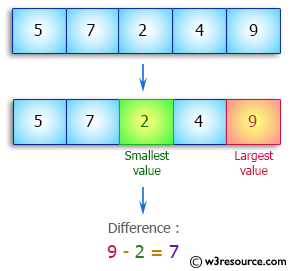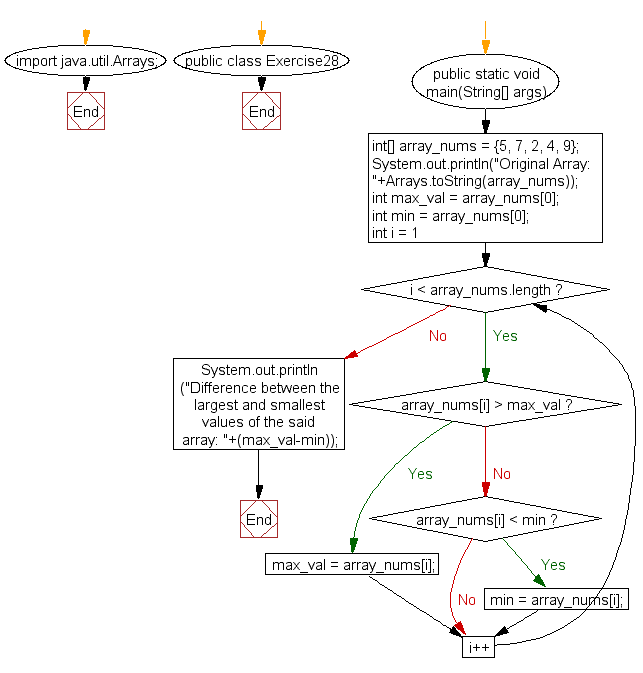﻿ Java: Difference of largest and smallest values in an array# Java Array Exercises: Get the difference between the largest and smallest values in an array of integers

## Java Array: Exercise-28 with Solution

Write a Java program to get the difference between the largest and smallest values in an array of integers. The length of the array must be 1 and above.

Pictorial Presentation:Sample Solution:

Java Code:

``````import java.util.Arrays;
public class Exercise28 {
public static void main(String[] args)
{
int[] array_nums = {5, 7, 2, 4, 9};
System.out.println("Original Array: "+Arrays.toString(array_nums));
int max_val = array_nums;
int min = array_nums;
for(int i = 1; i < array_nums.length; i++)
{
if(array_nums[i] > max_val)
max_val = array_nums[i];
else if(array_nums[i] < min)
min = array_nums[i];
}
System.out.println("Difference between the largest and smallest values of the said array: "+(max_val-min));
}
}
```
```

Sample Output:

```
Original Array: [5, 7, 2, 4, 9]
Difference between the largest and smallest values of the said array: 7
```

Flowchart:Visualize Java code execution (Python Tutor):

Java Code Editor:

Improve this sample solution and post your code through Disqus

What is the difficulty level of this exercise?

Test your Programming skills with w3resource's quiz.

﻿

## Java: Tips of the Day

countOccurrences

Counts the occurrences of a value in an array.

Use Arrays.stream().filter().count() to count total number of values that equals the specified value.

```public static long countOccurrences(int[] numbers, int value) {
return Arrays.stream(numbers)
.filter(number -> number == value)
.count();
}
```

Ref: https://bit.ly/3kCAgLb